Normal view MARC view ISBD view

# Ordinary differential equations : principles and applications / A.K. Nandakumaran, P.S. Datti, Raju K. George.

Material type:TextSeries: Cambridge-IISc series.Publisher: Cambridge ; New York, NY : Cambridge University Press, 2017Description: xviii, 328 pages : Rs. 595.00 [accession number 10763 gifted by NBHM] illustrations ; 24 cm.Content type: text Media type: unmediated Carrier type: volumeISBN: 9781108416412 (hardback : alk. paper).Subject(s): Differential equationsDDC classification: 515/.352 Summary: "Discusses the relevant topics including first and second order linear equations, initial value problems and qualitative theory"-- Provided by publisher.
List(s) this item appears in: 2019-07-04
Item type Current location Call number Status Date due Barcode Item holds
Book Chennai Mathematical Institute
General Stacks
515.352 NAN (Browse shelf) Available 10763
Book Chennai Mathematical Institute
General Stacks
515.352 NAN (Browse shelf) Available 10668
Total holds: 0
##### Browsing Chennai Mathematical Institute Shelves , Shelving location: General Stacks Close shelf browser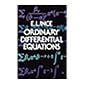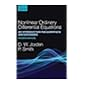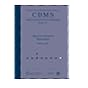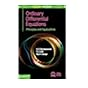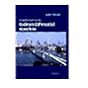515.352 INC Ordinary differential equations. 515.352 JOR Nonlinear ordinary differential equations ; an introduction for scientists and engineers / 515.352 JOS Discrete Painleve equations / 515.352 NAN Ordinary differential equations : principles and applications / 515.352 NAN Ordinary differential equations : principles and applications / 515.352 ROB An introduction to ordinary differential equations / 515.352 TES Ordinary differential equations and dynamical systems /

Includes bibliographical references and index.

"Discusses the relevant topics including first and second order linear equations, initial value problems and qualitative theory"-- Provided by publisher.